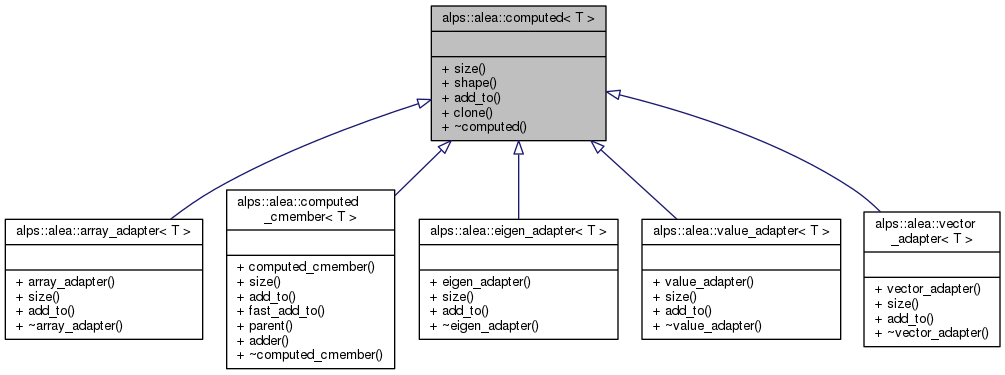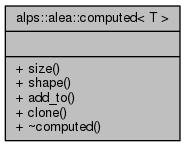ALPSCore reference
alps::alea::computed< T > Struct Template Referenceabstract

#include <core.hpp>

Inheritance diagram for alps::alea::computed< T >:Collaboration diagram for alps::alea::computed< T >:## Public Types

typedef T value_type

## Public Member Functions

virtual size_t size () const =0

virtual std::vector< size_t > shape () const

virtual void add_to (view< T > out) const =0

virtual computedclone ()

virtual ~computed ()

## Detailed Description

### template<typename T> struct alps::alea::computed< T >

Interface for a computed result (a result computed on-the-fly).

As a trivial example, here is a vector-valued estimator of size 2 that always adds the vector [1.0, -1.0] to the buffer:

struct trivial_computed : public computed<double>
{
size_t size() const { return 2; }
void add_to(view<T> out) { out += 1.0; out -= 1.0; }
}


If a computed is passed to an accumulator, the accumulator will call the add_to() method zero or more times with different buffers. This allows to avoid temporaries, as the addend can be constructed in-place, which also allows for sparse data. Also, as there is usually a bin size > 1, adding is the fundamental operation.

See also: computed_wrapper<T>

Definition at line 157 of file core.hpp.

## Member Typedef Documentation

template<typename T>
 typedef T alps::alea::computed< T >::value_type

Definition at line 159 of file core.hpp.

## Constructor & Destructor Documentation

template<typename T>
 virtual alps::alea::computed< T >::~computed ( )
inlinevirtual

Destroy estimator

Definition at line 180 of file core.hpp.

## Member Function Documentation

template<typename T>
 virtual void alps::alea::computed< T >::add_to ( view< T > out ) const
pure virtual

Add computed result data to the buffer in out. If in(i) is the i-th component of the estimator, do the equivalent of:

for (size_t i = 0; i != size(); ++i)
out[i] += in(i);
template<typename T>
 virtual computed* alps::alea::computed< T >::clone ( )
inlinevirtual

Returns a clone of the estimator (optional)

Definition at line 177 of file core.hpp.

template<typename T>
 virtual std::vector alps::alea::computed< T >::shape ( ) const
inlinevirtual

Return the shape of the data - product must equal size

Definition at line 165 of file core.hpp.

template<typename T>
 virtual size_t alps::alea::computed< T >::size ( ) const
pure virtual

Number of elements of the computed result

The documentation for this struct was generated from the following file: